# Regularity of global solution to atmospheric circulation equations with humidity effect

## Abstract

In this article, the regularity of the global solutions to atmospheric circulation equations with humidity effect is considered. Firstly, the formula of the global solutions is obtained by using the theory of linear operator semigroups. Secondly, the regularity of the global solutions to atmospheric circulation equations is presented by using mathematical induction and regularity estimates for the linear semigroups.

MSC:35D35, 35K20, 35Q35.

## 1 Introduction

This paper is concerned with the regularity of solutions to the following initial-boundary problem of atmospheric circulation equations involving unknown functions $\left(u,T,q,p\right)$ at $\left(x,t\right)=\left({x}_{1},{x}_{2},t\right)\in \mathrm{\Omega }×\left(0,\mathrm{\infty }\right)$ ($\mathrm{\Omega }=\left(0,2\pi \right)×\left(0,1\right)$ is a period of ${C}^{\mathrm{\infty }}$ field $\left(-\mathrm{\infty },+\mathrm{\infty }\right)×\left(0,1\right)$):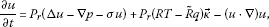(1.1)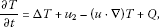(1.2)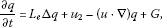(1.3)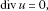(1.4)

where ${P}_{r}>0$, $R>0$, $\stackrel{˜}{R}$, ${L}_{e}>0$ are constants, $u=\left({u}_{1},{u}_{2}\right)$, T, q, p denote velocity field, temperature, humidity and pressure respectively, Q, G are known functions, and σ is a constant matrix

$\sigma =\left(\begin{array}{cc}{\sigma }_{0}& \omega \\ \omega & {\sigma }_{1}\end{array}\right).$

The problems (1.1)-(1.4) are supplemented with the following Dirichlet boundary condition at ${x}_{2}=0,1$ and the periodic condition for ${x}_{1}$: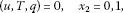(1.5)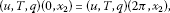(1.6)

and initial value conditions

$\left(u,T,q\right)=\left({u}_{0},{T}_{0},{q}_{0}\right),\phantom{\rule{1em}{0ex}}t=0.$
(1.7)

Partial differential equations (1.1)-(1.7) are presented in atmospheric circulation with humidity effect . Atmospheric circulation is one of the main factors affecting the global climate so it is very necessary to understand and master its mysteries and laws. Atmospheric circulation is an important mechanism to complete the transports and balance of atmospheric heat and moisture and the conversion between various energies. Moreover, it is also the important result of these physical transports, balance and conversion. Thus, it is of necessity to study the characteristics, formation, preservation, change and effects of the atmospheric circulation and master its evolution law, which is not only the essential part of human’s understanding of nature, but also a helpful method of changing and improving the accuracy of weather forecasts, exploring global climate change, and making effective use of climate resources.

The atmosphere and ocean around the earth are rotating geophysical fluids, which are also two important components of the climate system. The phenomena of the atmosphere and ocean are extremely rich in their organization and complexity, and a lot of them cannot be produced by laboratory experiments. The atmosphere or the ocean or the couple atmosphere and ocean can be viewed as initial and boundary value problems , or an infinite dimensional dynamical system . We deduce atmospheric circulation models which are able to show the features of atmospheric circulation and are easy to be studied from the very complex atmospheric circulation model based on the actual background and meteorological data, and we present global solutions of atmospheric circulation equations with the use of the T-weakly continuous operator . In , the steady state solutions to atmospheric circulation equations with humidity effect are studied. A sufficient condition of the existence of the steady state solutions to atmospheric circulation equations is obtained, and the regularity of the steady state solutions is verified. In this article, we investigate the regularity of the solutions to atmospheric circulation equations (1.1)-(1.7).

The paper is organized as follows. In Section 2, we present preliminary results. In Section 3, we present the formula of the solution to the atmospheric circulation equations. In Section 4, we obtain the regularity of the solutions to equations (1.1)-(1.7).

${\parallel \cdot \parallel }_{X}$ denotes the norm of the space X, and C, ${C}_{i}$ are variable constants.

## 2 Preliminaries

We consider the divergence form of the linear elliptic equation

$Lu=-{D}_{j}\left({a}_{ij}{D}_{i}u\right)+{b}_{i}{D}_{i}u+cu=f,$
(2.1)

where ${a}_{ij},{b}_{i},c\in {L}^{\mathrm{\infty }}\left(\mathrm{\Omega }\right)$, $f\in {L}^{2}\left(\mathrm{\Omega }\right)$, ${a}_{ij}={a}_{ji}$, $\left({a}_{ij}\right)$ is uniformly elliptic, i.e., there exist constants $0<{\lambda }_{1}\le {\lambda }_{2}$ such that

${\lambda }_{1}{|\xi |}^{2}\le {a}_{ij}\left(x\right){\xi }_{i}{\xi }_{j}\le {\lambda }_{2}{|\xi |}^{2},\phantom{\rule{1em}{0ex}}\mathrm{\forall }\xi \in {R}^{n},x\in \mathrm{\Omega }.$

The problem (2.1) is supplemented with the following Dirichlet boundary condition:

$u{|}_{\partial \mathrm{\Omega }}=\phi .$
(2.2)

Lemma 2.1  (Theory of linear elliptic equations)

Let $\mathrm{\Omega }\subset {R}^{n}$ be a ${C}^{2}$ field, ${a}_{ij}\in {C}^{0}\left(\mathrm{\Omega }\right)$, ${b}_{i},c\in {L}^{\mathrm{\infty }}\left(\mathrm{\Omega }\right)$, $f\in {L}^{p}\left(\mathrm{\Omega }\right)$, $\phi \in {W}^{2,p}\left(\mathrm{\Omega }\right)$. If $u\in {W}^{2,p}$ is a solution of Eqs. (2.1), (2.2), then

${\parallel u\parallel }_{{W}^{2,p}}\le C\left({\parallel u\parallel }_{{L}^{p}}+{\parallel f\parallel }_{{L}^{p}}+{\parallel \phi \parallel }_{{W}^{2,p}}\right),$

where $C>0$ depends on n, p, λ, Ω and ${C}^{0,\alpha }$-norm or ${L}^{\mathrm{\infty }}$-norm of the coefficient functions.

We consider the Stokes equation

$\left\{\begin{array}{c}-\mu \mathrm{△}u+\mathrm{\nabla }p=f\left(x\right),\hfill \\ divu=0,\hfill \\ u{|}_{\partial \mathrm{\Omega }}=\phi .\hfill \end{array}$
(2.3)

Lemma 2.2 [11, 12] (ADN theory of the Stokes equation)

Let $f\in {W}^{k,p}\left(\mathrm{\Omega },{R}^{n}\right)$, $\phi \in {W}^{k+2,p}\left(\mathrm{\Omega },{R}^{n}\right)$, $k\ge 0$. If $\left(u,p\right)\in {W}^{2,p}\left(\mathrm{\Omega },{R}^{n}\right)×{W}^{1,p}\left(\mathrm{\Omega }\right)$ ($1) is a solution of Eq. (2.3), then the solution $\left(u,p\right)\in {W}^{k+2,p}\left(\mathrm{\Omega },{R}^{n}\right)×{W}^{k+1,p}\left(\mathrm{\Omega }\right)$, and

${\parallel u\parallel }_{{W}^{k+2,p}}+{\parallel p\parallel }_{{W}^{k+1,p}}\le C\left({\parallel f\parallel }_{{W}^{k,p}}+{\parallel \left(u,p\right)\parallel }_{{L}^{p}}+{\parallel \phi \parallel }_{{W}^{k+2,p}}\right),$

where $C>0$ depends on μ, n, k, α, Ω.

Let X be a linear space, ${X}_{1}$, ${X}_{2}$ be two separable reflexive Banach spaces, and H be a Hilbert space. ${X}_{1}$, ${X}_{2}$, and H are completion spaces of X under the respective norm. ${X}_{1},{X}_{2}\subset H$ are dense embedding. $F:{X}_{2}×\left(0,\mathrm{\infty }\right)\to {X}_{1}^{\ast }$ is a continuous mapping. We consider the abstract equation

$\left\{\begin{array}{cc}\frac{du}{dt}=Fu,\hfill & 0
(2.4)

where $\phi \in H$, $u:\left[0,+\mathrm{\infty }\right)\to H$ is unknown.

Definition 2.3 Let $\phi \in H$ be a given initial value. $u\in {L}^{p}\left(\left(0,T\right),{X}_{2}\right)\cap {L}^{\mathrm{\infty }}\left(\left(0,T\right),H\right)$ ($0) is called a global solution of Eq. (2.4) if u satisfies

${\left(u\left(t\right),v\right)}_{H}={\int }_{0}^{t}〈Fu,v〉\phantom{\rule{0.2em}{0ex}}dt+{\left(\phi ,v\right)}_{H},\phantom{\rule{1em}{0ex}}\mathrm{\forall }v\in {X}_{1}\subset H.$

Definition 2.4 Let ${u}_{n},{u}_{0}\in {L}^{p}\left(\left(0,T\right),{X}_{2}\right)$. ${u}_{n}\to {u}_{0}$ is called uniformly weak convergence in ${L}^{p}\left(\left(0,T\right),{X}_{2}\right)$ if $\left\{{u}_{n}\right\}\subset {L}^{\mathrm{\infty }}\left(\left(0,T\right),H\right)$ is bounded, and

(2.5)

Definition 2.5 A mapping $F:{X}_{2}×\left(0,\mathrm{\infty }\right)\to {X}_{1}^{\ast }$ is called T-weakly continuous if for $p=\left({p}_{1},\dots ,{p}_{m}\right)$, $0 and ${u}_{n}$ uniformly weakly converges to ${u}_{0}$, we have

$\underset{n\to \mathrm{\infty }}{lim}{\int }_{0}^{T}〈F{u}_{n},v〉\phantom{\rule{0.2em}{0ex}}dt={\int }_{0}^{T}〈F{u}_{0},v〉\phantom{\rule{0.2em}{0ex}}dt,\phantom{\rule{1em}{0ex}}\mathrm{\forall }v\in {X}_{1}.$

Lemma 2.6 

Assume $F:{X}_{2}×\left(0,\mathrm{\infty }\right)\to {X}_{1}^{\ast }$ is T-weakly continuous and satisfies:

(A1) there exists $p=\left({p}_{1},\dots ,{p}_{m}\right)$, ${p}_{i}>1$ ($1\le i\le m$), such that

$〈Fu,u〉\le -{C}_{1}{\parallel u\parallel }_{{X}_{2}}^{p}+{C}_{2}{\parallel u\parallel }_{H}^{2}+f\left(t\right),\phantom{\rule{1em}{0ex}}\mathrm{\forall }u\in X,$

where ${C}_{1}$, ${C}_{2}$ are constants, $f\in {L}^{1}\left(0,T\right)$ ($0), ${\parallel \cdot \parallel }_{{X}_{2}}^{p}={\sum }_{i=1}^{m}{|\cdot |}_{i}^{{p}_{i}}$, ${|\cdot |}_{i}$ is a seminorm of ${X}_{2}$, ${\parallel \cdot \parallel }_{{X}_{2}}={\sum }_{i=1}^{m}{|\cdot |}_{i}$,

(A2) there exists $0<\alpha <1$ for any $0 and $u\in {C}^{1}\left(\left[0,\mathrm{\infty }\right),X\right)$,

$|{\int }_{t}^{t+h}〈Fu,v〉\phantom{\rule{0.2em}{0ex}}dt|\le C{h}^{\alpha },\phantom{\rule{1em}{0ex}}\mathrm{\forall }v\in X\phantom{\rule{0.5em}{0ex}}\mathit{\text{and}}\phantom{\rule{0.5em}{0ex}}0\le t

where $C>0$ depends only on T, ${\parallel v\parallel }_{{X}_{1}}$, ${\int }_{0}^{t}{\parallel u\parallel }_{{X}_{2}}^{p}\phantom{\rule{0.2em}{0ex}}dt$, and ${sup}_{0\le t\le T}{\parallel u\parallel }_{H}$.

Then for any $\phi \in H$, Eq. (2.4) has a global weak solution

If $F:{X}_{2}×\left(0,\mathrm{\infty }\right)\to {X}_{1}^{\ast }$ is Frechét differentiable, then the regular solution can be presented under some condition.

We introduce a space sequence

$X\subset \stackrel{˜}{H}\subset {X}_{3}\subset {X}_{1}\subset {H}_{1}\subset H,$

where X, ${X}_{1}$, H are such as in Lemma 2.6, ${X}_{3}$ is a Banach space, ${H}_{1}$ is a Hilbert space, and ${H}_{1}\subset H$ are compact including. There exist a constant ${C}_{1}\ge 0$ and a nonnegative function $\alpha \in {L}^{1}\left(0,T\right)$ ($0) such that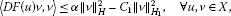(2.6)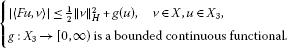(2.7)

Lemma 2.7 In addition to the assumptions about the existence of a global solution in Lemma  2.6, if $F:{X}_{2}×\left(0,\mathrm{\infty }\right)\to {X}_{1}^{\ast }$ is Frechét differentiable and satisfies (2.6), (2.7), then Eq. (2.4) has a unique global solution

$u\in {W}^{1,\mathrm{\infty }}\left(\left(0,T\right),H\right)\cap {W}^{1,2}\left(\left(0,T\right),{H}_{1}\right),\phantom{\rule{1em}{0ex}}0

for all $\phi \in {X}_{3}$.

Lemma 2.8 

Let L be a generator of a strongly continuous semigroup $\mathrm{\Phi }\left(t\right)$. If $u\left(\cdot ,\phi \right)\in {L}^{1}\left(\left(0,T\right),Y\right)$ is a weak solution to the equation

$\left\{\begin{array}{c}\frac{du}{dt}=Lu+F\left(u\right),\phantom{\rule{1em}{0ex}}0
(2.8)

and $F\left(u\left(\cdot ,\phi \right)\right)\in {L}^{1}\left(\left(0,T\right),X\right)$, then the solution $u\left(t,\phi \right)$ can be read as

$u=\mathrm{\Phi }\left(t\right)\phi +{\int }_{0}^{t}\mathrm{\Phi }\left(t-\tau \right)F\left(u\right)\phantom{\rule{0.2em}{0ex}}d\tau ,\phantom{\rule{1em}{0ex}}0\le t\le T.$

Note that we used to assume that the linear operator L in (2.8) is a sectorial operator which generates an analytic semigroup $\mathrm{\Phi }\left(t\right)$. It is known that there exists a constant $\lambda \ge 0$ such that $L-\lambda I$ generates the fractional power operators ${\mathcal{L}}^{\alpha }$ and fractional order spaces ${H}_{\alpha }$ for $\alpha \in {R}^{1}$, where $\mathcal{L}=-\left(L-\lambda I\right)$. Without loss of generality, we assume that generates the fractional power operators ${\mathcal{L}}^{\alpha }$ and fractional order spaces ${H}_{\alpha }$ as follows:

${\mathcal{L}}^{\alpha }={\left(-L\right)}^{\alpha }:{H}_{\alpha }\to H,\phantom{\rule{1em}{0ex}}\alpha \in {R}^{1},$

where ${H}_{\alpha }=D\left({\mathcal{L}}^{\alpha }\right)$ is the domain of ${\mathcal{L}}^{\alpha }$. By the semigroup theory of linear operators (Pazy ), we know that ${H}_{\beta }\subset {H}_{\alpha }$ is a compact inclusion for any $\beta >\alpha$.

Lemma 2.9  (Imbedding theorem of factional order spaces)

Let $\mathrm{\Omega }\subset {R}^{n}$ be a Lipschitz field, $L:{W}^{m,p}\to {L}^{p}\left(\mathrm{\Omega }\right)$ be a sectorial operator, $m\ge 2$, and $1\le p<\mathrm{\infty }$. Then for $0\le \alpha \le 1$, the fractional order spaces ${H}_{\alpha }=D\left({\mathcal{L}}^{\alpha }\right)$ satisfy the following relations: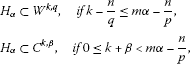and the inequalities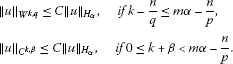For sectorial operators, we also have the following properties which can be found in .

Lemma 2.10 Let $L:{H}_{1}\to H$ be a sectorial operator which generates an analytic semigroup $T\left(t\right)={e}^{tL}$. If all eigenvalues λ of L satisfy $Re\lambda <-{\lambda }_{0}$ for some real number ${\lambda }_{0}>0$, then for ${\mathcal{L}}^{\alpha }\left(\mathcal{L}=-L\right)$, we have

1. (1)

$T\left(t\right):H\to {H}_{\alpha }$ is bounded for all $\alpha \in {R}^{1}$ and $t>0$,

2. (2)

$T\left(t\right){\mathcal{L}}^{\alpha }x={\mathcal{L}}^{\alpha }T\left(t\right)x$, $\mathrm{\forall }x\in {H}_{\alpha }$,

3. (3)

for each $t>0$, ${\mathcal{L}}^{\alpha }T\left(t\right):H\to H$ is bounded and

$\parallel {\mathcal{L}}^{\alpha }T\left(t\right)\parallel \le {C}_{\alpha }{t}^{-\alpha }{e}^{-\delta t},$

where some $\delta >0$, ${C}_{\alpha }>0$ is a constant only depending on α,

1. (4)

the ${H}_{\alpha }$-norm can be defined by

${\parallel x\parallel }_{{H}_{\alpha }}={\parallel {\mathcal{L}}^{\alpha }x\parallel }_{H},$
(2.9)
2. (5)

if is symmetric, for any $\alpha ,\beta \in {R}^{1}$, we have

${〈{\mathcal{L}}^{\alpha }u,v〉}_{H}={〈{\mathcal{L}}^{\alpha -\beta }u,{\mathcal{L}}^{\beta }v〉}_{H}.$

## 3 Formula of global solutions

We introduce the spacesLet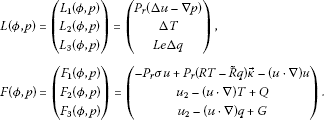Then Eqs. (1.1)-(1.7) can be rewritten as an abstract equation

$\frac{d\varphi }{dt}=L\varphi +F\left(\varphi \right).$

Theorem 3.1 If ${\varphi }_{0}=\left({u}_{0},{T}_{0},{q}_{0}\right)\in H$, $Q,G\in {L}^{2}\left(\mathrm{\Omega }\right)$, then the global solution ϕ of Eqs. (1.1)-(1.7) can be read as

$\varphi \left(x,t\right)=\mathrm{\Phi }\left(t\right){\varphi }_{0}+{\int }_{0}^{t}\mathrm{\Phi }\left(t-\tau \right)P\left(F\left(\varphi \right)\right)\phantom{\rule{0.2em}{0ex}}d\tau ,$
(3.1)

where $\mathrm{\Phi }\left(t\right)$ is an analytic semigroup generated by L, and $P:{L}^{2}\left(\mathrm{\Omega },{R}^{4}\right)\to H$ is a Leray projection.

Proof As $\varphi =\left(u,T,q\right)\in {L}^{\mathrm{\infty }}\left(\left(0,T\right),H\right)\cap {L}^{2}\left(\left(0,T\right),{H}_{1}\right)$ is a weak solution to Eqs. (1.1)-(1.7) , from the Hölder inequality and the Sobolev imbedding theorem, it follows that

$\begin{array}{rcl}{\int }_{0}^{T}{\left[{\int }_{\mathrm{\Omega }}{|\left(u\cdot \mathrm{\nabla }\right)u|}^{\frac{3}{2}}\phantom{\rule{0.2em}{0ex}}dx\right]}^{\frac{2}{3}}\phantom{\rule{0.2em}{0ex}}dt& \le & {\int }_{0}^{T}{\left[{\int }_{\mathrm{\Omega }}{|u|}^{\frac{3}{2}}{|\mathrm{\nabla }u|}^{\frac{3}{2}}\phantom{\rule{0.2em}{0ex}}dx\right]}^{\frac{2}{3}}\phantom{\rule{0.2em}{0ex}}dt\\ \le & {\int }_{0}^{T}\left[{\left({\int }_{\mathrm{\Omega }}{|u|}^{6}\phantom{\rule{0.2em}{0ex}}dx\right)}^{\frac{1}{6}}{\left({\int }_{\mathrm{\Omega }}{|Du|}^{2}\phantom{\rule{0.2em}{0ex}}dx\right)}^{\frac{1}{2}}\right]\phantom{\rule{0.2em}{0ex}}dt\\ \le & C{\int }_{0}^{T}{\int }_{\mathrm{\Omega }}{|Du|}^{2}\phantom{\rule{0.2em}{0ex}}dx\phantom{\rule{0.2em}{0ex}}dt\le C{\parallel u\parallel }_{{L}^{2}\left(0,T;{H}^{1}\right)}^{2}.\end{array}$

Then $\left(u\cdot \mathrm{\nabla }\right)u\in {L}^{1}\left(\left(0,T\right),{L}^{\frac{3}{2}}\left(\mathrm{\Omega }\right)\right)$. Hence,

$-{P}_{r}\sigma u+{P}_{r}\left(RT-\stackrel{˜}{R}q\right)\stackrel{\to }{\kappa }-\left(u\cdot \mathrm{\nabla }\right)u\in {L}^{1}\left(\left(0,T\right),{L}^{\frac{3}{2}}\left(\mathrm{\Omega }\right)\right).$
(3.2)

From the Hölder inequality and the Sobolev imbedding theorem, we see

$\begin{array}{rcl}{\int }_{0}^{T}{\left[{\int }_{\mathrm{\Omega }}{|\left(u\cdot \mathrm{\nabla }\right)T|}^{\frac{3}{2}}\phantom{\rule{0.2em}{0ex}}dx\right]}^{\frac{2}{3}}\phantom{\rule{0.2em}{0ex}}dt& \le & {\int }_{0}^{T}{\left[{\int }_{\mathrm{\Omega }}{|u|}^{\frac{3}{2}}{|DT|}^{\frac{3}{2}}\phantom{\rule{0.2em}{0ex}}dx\right]}^{\frac{2}{3}}\phantom{\rule{0.2em}{0ex}}dt\\ \le & {\int }_{0}^{T}\left[{\left({\int }_{\mathrm{\Omega }}{|u|}^{6}\phantom{\rule{0.2em}{0ex}}dx\right)}^{\frac{1}{6}}{\left({\int }_{\mathrm{\Omega }}{|DT|}^{2}\phantom{\rule{0.2em}{0ex}}dx\right)}^{\frac{1}{2}}\right]\phantom{\rule{0.2em}{0ex}}dt\\ \le & C{\int }_{0}^{T}\left[{\left({\int }_{\mathrm{\Omega }}{|Du|}^{2}\phantom{\rule{0.2em}{0ex}}dx\right)}^{\frac{1}{2}}{\left({\int }_{\mathrm{\Omega }}{|DT|}^{2}\phantom{\rule{0.2em}{0ex}}dx\right)}^{\frac{1}{2}}\right]\phantom{\rule{0.2em}{0ex}}dt\\ \le & C{\left({\int }_{0}^{T}{\int }_{\mathrm{\Omega }}{|Du|}^{2}\phantom{\rule{0.2em}{0ex}}dx\phantom{\rule{0.2em}{0ex}}dt\right)}^{\frac{1}{2}}{\left({\int }_{0}^{T}{\int }_{\mathrm{\Omega }}{|DT|}^{2}\phantom{\rule{0.2em}{0ex}}dx\phantom{\rule{0.2em}{0ex}}dt\right)}^{\frac{1}{2}}\\ \le & C{\parallel T\parallel }_{{L}^{2}\left(0,T;{H}^{1}\right)}{\parallel u\parallel }_{{L}^{2}\left(0,T;{H}^{1}\right)}.\end{array}$

Then $\left(u\cdot \mathrm{\nabla }\right)T\in {L}^{1}\left(\left(0,T\right),{L}^{\frac{3}{2}}\left(\mathrm{\Omega }\right)\right)$. Thus,

${u}_{2}-\left(u\cdot \mathrm{\nabla }\right)T+Q\in {L}^{1}\left(\left(0,T\right),{L}^{\frac{3}{2}}\left(\mathrm{\Omega }\right)\right).$
(3.3)

Similarly, we have

${u}_{2}-\left(u\cdot \mathrm{\nabla }\right)q+G\in {L}^{1}\left(\left(0,T\right),{L}^{\frac{3}{2}}\left(\mathrm{\Omega }\right)\right).$
(3.4)

According to the ADN theory and the theory of linear elliptic equations, we have that

$L\left(\varphi ,p\right)=\left(\begin{array}{c}{P}_{r}\left(\mathrm{\Delta }u-\mathrm{\nabla }p\right)\\ \mathrm{\Delta }T\\ {L}_{e}\mathrm{\Delta }q\end{array}\right)$

is a sectorial operator and

$L:{W}^{2,q}\left(\mathrm{\Omega },{R}^{4}\right)×{W}^{1,q}\left(\mathrm{\Omega }\right)\to {L}^{q}\left(\mathrm{\Omega },{R}^{4}\right),\phantom{\rule{1em}{0ex}}\mathrm{\forall }1

Therefore, L generates the analytic semigroup $\mathrm{\Phi }\left(t\right)$.

It follows from (3.2), (3.3), and (3.4) that

$F\left(\varphi \right)\in {L}^{1}\left(\left(0,T\right),{L}^{\frac{3}{2}}\left(\mathrm{\Omega },{R}^{4}\right)\right).$

Applying Lemma 2.8 yields

$\varphi \left(x,t\right)=\mathrm{\Phi }\left(t\right){\varphi }_{0}+{\int }_{0}^{t}\mathrm{\Phi }\left(t-\tau \right)P\left(F\left(\varphi \right)\right)\phantom{\rule{0.2em}{0ex}}d\tau .$

□

Remark 3.2 The analytic semigroup $\mathrm{\Phi }\left(t\right)$ generated by L can be read as

$\mathrm{\Phi }\left(t\right)=\left(\begin{array}{c}{\mathrm{\Phi }}_{1}\left(t\right)\\ {\mathrm{\Phi }}_{2}\left(t\right)\\ {\mathrm{\Phi }}_{3}\left(t\right)\end{array}\right).$

Remark 3.3 The semigroup generated by Eqs. (1.1)-(1.7) can be rewritten as

$\left(\begin{array}{c}u\\ T\\ q\end{array}\right)=\left(\begin{array}{c}{\mathrm{\Phi }}_{1}\left(t\right)\left({\varphi }_{0}\right)+{\int }_{0}^{t}{\mathrm{\Phi }}_{1}\left(t-\tau \right)P\left({F}_{1}\right)\phantom{\rule{0.2em}{0ex}}d\tau \\ {\mathrm{\Phi }}_{2}\left(t\right)\left({\varphi }_{0}\right)+{\int }_{0}^{t}{\mathrm{\Phi }}_{2}\left(t-\tau \right)P\left({F}_{2}\right)\phantom{\rule{0.2em}{0ex}}d\tau \\ {\mathrm{\Phi }}_{3}\left(t\right)\left({\varphi }_{0}\right)+{\int }_{0}^{t}{\mathrm{\Phi }}_{3}\left(t-\tau \right)P\left({F}_{3}\right)\phantom{\rule{0.2em}{0ex}}d\tau \end{array}\right).$

## 4 Regularity of global solution

Theorem 4.1 If ${\varphi }_{0}=\left({u}_{0},{T}_{0},{q}_{0}\right)\in {H}^{2}\left(\mathrm{\Omega },{R}^{4}\right)\cap {H}_{1}$, $Q,G\in {L}^{2}\left(\mathrm{\Omega }\right)$, then Eqs. (1.1)-(1.7) have a unique solution $\left(\varphi ,p\right)=\left(u,T,q,p\right)$, and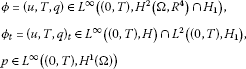for all $0.

Proof Let ${X}_{2}={X}_{1}={H}_{1}$ and ${X}_{3}={H}^{2}\left(\mathrm{\Omega },{R}^{4}\right)\cap {H}_{1}$. Define $F:{H}_{1}\to {H}_{1}^{\ast }$ as

$\begin{array}{rcl}〈F\varphi ,\psi 〉& =& {\int }_{\mathrm{\Omega }}\left[-{P}_{r}\mathrm{\nabla }u\mathrm{\nabla }v-{P}_{r}\sigma u\cdot u+{P}_{r}\left(RT-\stackrel{˜}{R}q\right){v}_{2}-\left(u\cdot \mathrm{\nabla }\right)u\cdot v-\mathrm{\nabla }T\mathrm{\nabla }S\\ +{u}_{2}S-\left(u\cdot \mathrm{\nabla }\right)TS+QS-{L}_{e}\mathrm{\nabla }q\mathrm{\nabla }z+{u}_{2}z-\left(u\cdot \mathrm{\nabla }\right)qz+Gz\right]\phantom{\rule{0.2em}{0ex}}dx,\\ \mathit{\text{for}}\phantom{\rule{0.5em}{0ex}}\mathrm{\forall }\psi =\left(v,S,z\right)\in {H}_{1}.\end{array}$

Then

$\begin{array}{rcl}〈F\varphi ,\psi 〉& =& {\int }_{\mathrm{\Omega }}\left[{P}_{r}\mathrm{\Delta }u-{P}_{r}\sigma u+{P}_{r}\left(RT-\stackrel{˜}{R}q\right)\stackrel{˜}{\kappa }-\left(u\cdot \mathrm{\nabla }\right)u\right]v+\left[\mathrm{\Delta }T+{u}_{2}-\left(u\cdot \mathrm{\nabla }\right)T+Q\right]S\\ +\left[{L}_{e}\mathrm{\Delta }q+{u}_{2}-\left(u\cdot \mathrm{\nabla }\right)q+G\right]z\phantom{\rule{0.2em}{0ex}}dx\\ \le & \frac{1}{2}{\int }_{\mathrm{\Omega }}\left({|v|}^{2}+{|S|}^{2}+{|z|}^{2}\right)\phantom{\rule{0.2em}{0ex}}dx+C{\int }_{\mathrm{\Omega }}\left({|\mathrm{\Delta }u|}^{2}+{|T|}^{2}+{|q|}^{2}+{|u\cdot Du|}^{2}+{|\mathrm{\Delta }T|}^{2}\\ +{|{u}_{2}|}^{2}+{|u\cdot DT|}^{2}+{|Q|}^{2}+{|\mathrm{\Delta }q|}^{2}+{|u\cdot Dq|}^{2}+{|G|}^{2}\right)\phantom{\rule{0.2em}{0ex}}dx\\ \le & \frac{1}{2}{\parallel \psi \parallel }_{H}^{2}+g\left(\varphi \right)\end{array}$

for any $\psi \in {H}_{1}$ and $\varphi \in {X}_{3}$. Then (2.7) holds.

We prove (2.6).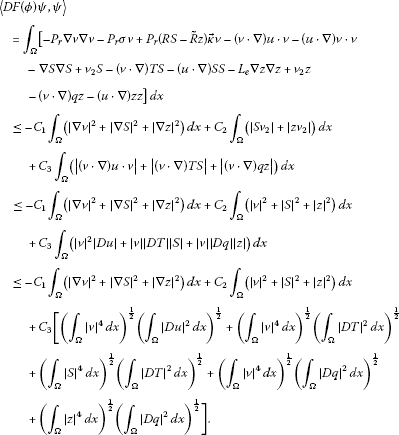By the interpolation inequality , we see

${\parallel v\parallel }_{{X}_{\frac{1}{4}}}={\parallel v\parallel }_{{H}^{\frac{1}{2}}}\le C{\parallel v\parallel }_{{L}^{2}}^{\frac{1}{2}}{\parallel v\parallel }_{{H}^{1}}^{\frac{1}{2}}.$
(4.1)

By the imbedding theorem of factional order spaces, we have

${\left({\int }_{\mathrm{\Omega }}{|v|}^{4}\phantom{\rule{0.2em}{0ex}}dx\right)}^{\frac{1}{4}}\le C{\parallel v\parallel }_{{X}_{\frac{1}{4}}}.$
(4.2)

Then it follows from (4.1) and 4.2) that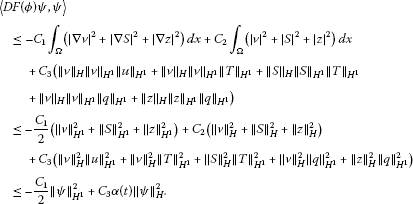Since $\varphi =\left(u,T,q\right)\in {L}^{2}\left(\left(0,T\right),{H}_{1}\right)$ is a weak solution to Eqs. (1.1)-(1.7), $\alpha \left(t\right)=C\left({\parallel u\parallel }_{{H}^{1}}^{2}+{\parallel T\parallel }_{{H}^{1}}^{2}+{\parallel q\parallel }_{{H}^{1}}^{2}+1\right)\in {L}^{1}\left(0,T\right)$, $0. Then (2.6) and (2.7) hold. From Lemma 2.7, we deduce that the solution ϕ is unique and

${\varphi }_{t}=\left({u}_{t},{T}_{t},{q}_{t}\right)\in {L}^{\mathrm{\infty }}\left(\left(0,T\right),H\right)\cap {L}^{2}\left(\left(0,T\right),{H}_{1}\right).$
(4.3)

Multiplying (1.1) by u and integrating over Ω, we get

${P}_{r}{\int }_{\mathrm{\Omega }}{|\mathrm{\nabla }u|}^{2}\phantom{\rule{0.2em}{0ex}}dx+{P}_{r}{\int }_{\mathrm{\Omega }}\sigma u\cdot u\phantom{\rule{0.2em}{0ex}}dx={\int }_{\mathrm{\Omega }}\left[{P}_{r}\left(RT-\stackrel{˜}{R}q\right)\stackrel{\to }{\kappa }u-{u}_{t}u\right]\phantom{\rule{0.2em}{0ex}}dx.$

Using the Young inequality, we obtain

${P}_{r}{\int }_{\mathrm{\Omega }}{|\mathrm{\nabla }u|}^{2}\phantom{\rule{0.2em}{0ex}}dx+{P}_{r}\stackrel{˜}{\sigma }{\int }_{\mathrm{\Omega }}{u}^{2}\phantom{\rule{0.2em}{0ex}}dx\le \epsilon {\int }_{\mathrm{\Omega }}{|u|}^{2}\phantom{\rule{0.2em}{0ex}}dx+C{\epsilon }^{-1}{\int }_{\mathrm{\Omega }}\left[{|T|}^{2}+{|q|}^{2}+{|{u}_{t}|}^{2}\right]\phantom{\rule{0.2em}{0ex}}dx,$

where $\epsilon >0$ is a real constant satisfying ${P}_{r}\stackrel{˜}{\sigma }\ge \epsilon$. Then there exists a constant $C>0$ such that

${\int }_{\mathrm{\Omega }}{|\mathrm{\nabla }u|}^{2}\phantom{\rule{0.2em}{0ex}}dx\le C\left({\parallel T\parallel }_{{L}^{2}}^{2}+{\parallel q\parallel }_{{L}^{2}}^{2}+{\parallel {u}_{t}\parallel }_{{L}^{2}}^{2}\right).$

Thanks to (4.3), we have

$u\in {L}^{\mathrm{\infty }}\left(\left(0,T\right),{H}^{1}\left(\mathrm{\Omega },{R}^{2}\right)\right).$
(4.4)

We consider the Stokes equation

$\left\{\begin{array}{c}-\mathrm{\Delta }u+\mathrm{\nabla }p=g\left(x,t\right),\phantom{\rule{1em}{0ex}}g=-\sigma u+\left(RT-\stackrel{˜}{R}q\right)\stackrel{\to }{\kappa }-\frac{1}{Pr}\left(u\cdot \mathrm{\nabla }\right)u-{u}_{t},\hfill \\ divu=0,\hfill \\ u=0,\phantom{\rule{1em}{0ex}}{x}_{2}=0,1,\hfill \\ u\left(0,{x}_{2}\right)=u\left(2\pi ,{x}_{2}\right).\hfill \end{array}$
(4.5)

From (4.3), (4.4), and the Sobolev imbedding theorem, we find that $g\left(x,t\right)\in {L}^{q}\left(\mathrm{\Omega },{R}^{2}\right)$, $\mathrm{\forall }1. By the ADN theorem, Eq. (4.5) has a solution

$u\in {L}^{\mathrm{\infty }}\left(\left(0,T\right),{W}^{2,q}\left(\mathrm{\Omega },{R}^{2}\right)\right).$

Then $\left(u\cdot \mathrm{\nabla }\right)u\in {L}^{2}\left(\mathrm{\Omega },{R}^{2}\right)$ and $g\left(x,t\right)\in {L}^{2}\left(\mathrm{\Omega },{R}^{2}\right)$. Using the ADN theorem, we obtain

$\left\{\begin{array}{c}u\in {L}^{\mathrm{\infty }}\left(\left(0,T\right),{H}^{2}\left(\mathrm{\Omega },{R}^{2}\right)\right),\hfill \\ p\in {L}^{\mathrm{\infty }}\left(\left(0,T\right),{H}^{1}\left(\mathrm{\Omega }\right)\right).\hfill \end{array}$
(4.6)

Multiplying (1.2) by T and integrating over Ω, we get

$\begin{array}{rcl}{\int }_{\mathrm{\Omega }}{|\mathrm{\nabla }T|}^{2}\phantom{\rule{0.2em}{0ex}}dx& =& {\int }_{\mathrm{\Omega }}\left({u}_{2}T+QT-{T}_{t}T\right)\phantom{\rule{0.2em}{0ex}}dx\\ \le & \epsilon {\int }_{\mathrm{\Omega }}{|T|}^{2}\phantom{\rule{0.2em}{0ex}}dx+C{\epsilon }^{-1}{\int }_{\mathrm{\Omega }}\left[{|u|}^{2}+{|Q|}^{2}+{|{T}_{t}|}^{2}\right]\phantom{\rule{0.2em}{0ex}}dx,\end{array}$

where $\epsilon >0$ is a constant. Then there exists a constant $C>0$ such that

${\int }_{\mathrm{\Omega }}{|\mathrm{\nabla }T|}^{2}\phantom{\rule{0.2em}{0ex}}dx\le C\left({\parallel u\parallel }_{{L}^{2}}^{2}+{\parallel Q\parallel }_{{L}^{2}}^{2}+{\parallel {T}_{t}\parallel }_{{L}^{2}}^{2}\right).$

Using (4.3), we have

$T\in {L}^{\mathrm{\infty }}\left(\left(0,T\right),{H}^{1}\right).$
(4.7)

We consider the elliptic equation

$\left\{\begin{array}{c}-\mathrm{\Delta }T={f}_{1},\phantom{\rule{1em}{0ex}}{f}_{1}={u}_{2}-\left(u\cdot \mathrm{\nabla }\right)T+Q-{T}_{t},\hfill \\ T=0,\phantom{\rule{1em}{0ex}}{x}_{2}=0,1,\hfill \\ T\left(0,{x}_{2}\right)=T\left(2\pi ,{x}_{2}\right).\hfill \end{array}$
(4.8)

It follows from (4.3), (4.7), and the Sobolev imbedding theorem that ${f}_{1}\left(x,t\right)\in {L}^{q}\left(\mathrm{\Omega }\right)$, $\mathrm{\forall }1. Using the theory of linear elliptic equations, Eq. (4.8) has a solution

$T\in {L}^{\mathrm{\infty }}\left(\left(0,T\right),{W}^{2,q}\left(\mathrm{\Omega }\right)\right).$

Then $\left(u\cdot \mathrm{\nabla }\right)T\in {L}^{2}\left(\mathrm{\Omega },{R}^{2}\right)$ and ${f}_{1}\left(x,t\right)\in {L}^{2}\left(\mathrm{\Omega },{R}^{2}\right)$. Using the theory of linear elliptic equations, we have that

$T\in {L}^{\mathrm{\infty }}\left(\left(0,T\right),{H}^{2}\left(\mathrm{\Omega }\right)\right).$
(4.9)

Multiplying (1.3) by q and integrating over Ω, we get

$\begin{array}{rcl}{\int }_{\mathrm{\Omega }}{|\mathrm{\nabla }q|}^{2}\phantom{\rule{0.2em}{0ex}}dx& =& {\int }_{\mathrm{\Omega }}\left({u}_{2}q+Gq-{q}_{t}\right)\phantom{\rule{0.2em}{0ex}}dx\\ \le & \epsilon {\int }_{\mathrm{\Omega }}{|q|}^{2}\phantom{\rule{0.2em}{0ex}}dx+C{\epsilon }^{-1}{\int }_{\mathrm{\Omega }}\left[{|u|}^{2}+{|G|}^{2}+{|{q}_{t}|}^{2}\right]\phantom{\rule{0.2em}{0ex}}dx,\end{array}$

where $\epsilon >0$ is a constant. Then there exists a constant $C>0$ such that

${\int }_{\mathrm{\Omega }}{|\mathrm{\nabla }q|}^{2}\phantom{\rule{0.2em}{0ex}}dx\le C\left({\parallel u\parallel }_{{L}^{2}}^{2}+{\parallel Q\parallel }_{{L}^{2}}^{2}+{\parallel {q}_{t}\parallel }_{{L}^{2}}^{2}\right).$

Using (4.3), we have

$q\in {L}^{\mathrm{\infty }}\left(\left(0,T\right),{H}^{1}\right).$
(4.10)

We consider the elliptic equation

$\left\{\begin{array}{c}-\mathrm{\Delta }q={f}_{2},\phantom{\rule{1em}{0ex}}{f}_{2}={u}_{2}-\left(u\cdot \mathrm{\nabla }\right)q+G-{q}_{t},\hfill \\ q=0,\phantom{\rule{1em}{0ex}}{x}_{2}=0,1,\hfill \\ q\left(2\pi ,{x}_{2}\right)=q\left(0,{x}_{2}\right).\hfill \end{array}$
(4.11)

Using the arguments similar to those for (4.8), we get

$q\in {L}^{\mathrm{\infty }}\left(\left(0,T\right),{H}^{2}\left(\mathrm{\Omega }\right)\right).$
(4.12)

It follows from (4.6), (4.9), and (4.12) that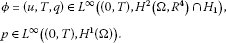□

Theorem 4.2 If ${\varphi }_{0}=\left({u}_{0},{T}_{0},{q}_{0}\right)\in {H}^{k+2}\left(\mathrm{\Omega },{R}^{4}\right)\cap {H}_{1}$, $Q,G\in {H}^{k}\left(\mathrm{\Omega }\right)$, then Eqs. (1.1)-(1.7) have a higher regular solution $\left(\varphi ,p\right)=\left(u,T,q,p\right)$ and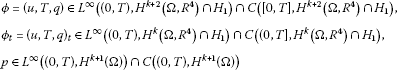for $\mathrm{\forall }0.

Proof We prove the theorem using mathematical induction.

If $k=1$, ${\varphi }_{0}=\left({u}_{0},{T}_{0},{q}_{0}\right)\in {H}^{3}\left(\mathrm{\Omega },{R}^{4}\right)\cap {H}_{1}$, $Q,G\in {H}^{1}\left(\mathrm{\Omega }\right)$, then ${\varphi }_{0}=\left({u}_{0},{T}_{0},{q}_{0}\right)\in {H}^{2}\left(\mathrm{\Omega },{R}^{4}\right)\cap {H}_{1}$, $Q,G\in {L}^{2}\left(\mathrm{\Omega }\right)$. Using Theorem 4.1, we find that $\varphi =\left(u,T,q\right)\in {H}^{2}\left(\mathrm{\Omega },{R}^{4}\right)$.

Thanks to the Sobolev imbedding theorem, ${H}^{2}\left(\mathrm{\Omega }\right)↪{W}^{1,4}\left(\mathrm{\Omega }\right)↪{C}^{0}\left(\mathrm{\Omega }\right)$ if $n=2$. We obtain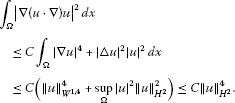Then $\left(u\cdot \mathrm{\nabla }\right)u\in {H}^{1}\left(\mathrm{\Omega }\right)$ and ${F}_{1}\left(\varphi \right)\in {H}^{1}\left(\mathrm{\Omega }\right)$.

We have from the formula (3.1)

${u}_{t}={L}_{1}{\mathrm{\Phi }}_{1}\left(t\right){u}_{0}+{\int }_{0}^{t}{L}_{1}{\mathrm{\Phi }}_{1}\left(t-\tau \right){F}_{1}\left(\mathrm{\Phi }\right)\phantom{\rule{0.2em}{0ex}}d\tau +{F}_{1}\left(\mathrm{\Phi }\right).$

Then there exists α satisfying $0<\alpha <1$ such that

$\begin{array}{rcl}{\parallel {u}_{t}\parallel }_{{H}^{1-\alpha }}& =& {\parallel {L}_{1}{\mathrm{\Phi }}_{1}\left(t\right){u}_{0}+{\int }_{0}^{t}{L}_{1}{\mathrm{\Phi }}_{1}\left(t-\tau \right){F}_{1}\left(\mathrm{\Phi }\right)\phantom{\rule{0.2em}{0ex}}d\tau +{F}_{1}\left(\mathrm{\Phi }\right)\parallel }_{{H}^{1-\alpha }}\\ \le & {\parallel {L}_{1}{\mathrm{\Phi }}_{1}\left(t\right){u}_{0}\parallel }_{{H}^{1-\alpha }}+{\parallel {\int }_{0}^{t}{L}_{1}{\mathrm{\Phi }}_{1}\left(t-\tau \right){F}_{1}\left(\mathrm{\Phi }\right)\phantom{\rule{0.2em}{0ex}}d\tau \parallel }_{{H}^{1-\alpha }}+{\parallel {F}_{1}\left(\mathrm{\Phi }\right)\parallel }_{{H}^{1-\alpha }}\\ \le & C+{\int }_{0}^{t}{\parallel {L}_{1}^{\frac{3-\alpha }{2}}{\mathrm{\Phi }}_{1}\left(t-\tau \right){F}_{1}\left(\mathrm{\Phi }\right)\parallel }_{{L}^{2}}\phantom{\rule{0.2em}{0ex}}d\tau \\ \le & C+{\int }_{0}^{t}\parallel {L}_{1}^{1-\frac{\alpha }{2}}{\mathrm{\Phi }}_{1}\left(t-\tau \right)\parallel {\parallel {L}_{1}^{\frac{1}{2}}{F}_{1}\left(\mathrm{\Phi }\right)\parallel }_{{L}^{2}}\phantom{\rule{0.2em}{0ex}}d\tau \le C.\end{array}$

Thus, ${u}_{t}\in {H}^{1-\alpha }\left(\mathrm{\Omega }\right)$. Then $g\in {H}^{1-\alpha }\left(\mathrm{\Omega }\right)$ in Eq. (4.5). By the ADN theory, $u\in {H}^{3-\alpha }\left(\mathrm{\Omega }\right)$. Thus, $\left(u\cdot \mathrm{\nabla }\right)u\in {H}^{2-\alpha }\left(\mathrm{\Omega }\right)$ and ${F}_{1}\left(\varphi \right)\in {H}^{2-\alpha }\left(\mathrm{\Omega }\right)$.

We have

$\begin{array}{rcl}{\parallel {u}_{t}\parallel }_{{H}^{1}}& =& {\parallel {L}_{1}{\mathrm{\Phi }}_{1}\left(t\right){u}_{0}+{\int }_{0}^{t}{L}_{1}{\mathrm{\Phi }}_{1}\left(t-\tau \right){F}_{1}\left(\mathrm{\Phi }\right)\phantom{\rule{0.2em}{0ex}}d\tau +{F}_{1}\left(\mathrm{\Phi }\right)\parallel }_{{H}^{1}}\\ \le & {\parallel {L}_{1}{\mathrm{\Phi }}_{1}\left(t\right){u}_{0}\parallel }_{{H}^{1}}+{\parallel {\int }_{0}^{t}{L}_{1}{\mathrm{\Phi }}_{1}\left(t-\tau \right){F}_{1}\left(\mathrm{\Phi }\right)\phantom{\rule{0.2em}{0ex}}d\tau \parallel }_{{H}^{1}}+{\parallel {F}_{1}\left(\mathrm{\Phi }\right)\parallel }_{{H}^{1}}\\ \le & C+{\int }_{0}^{t}{\parallel {L}_{1}^{\frac{3}{2}}{\mathrm{\Phi }}_{1}\left(t-\tau \right){F}_{1}\left(\mathrm{\Phi }\right)\parallel }_{{L}^{2}}\phantom{\rule{0.2em}{0ex}}d\tau \\ \le & C+{\int }_{0}^{t}\parallel {L}_{1}^{\frac{1+\alpha }{2}}{\mathrm{\Phi }}_{1}\left(t-\tau \right)\parallel {\parallel {L}_{1}^{1-\frac{\alpha }{2}}{F}_{1}\left(\mathrm{\Phi }\right)\parallel }_{{L}^{2}}\phantom{\rule{0.2em}{0ex}}d\tau \le C,\end{array}$

which implies ${u}_{t}\in {H}^{1}\left(\mathrm{\Omega }\right)$. Then $g\in {H}^{1}\left(\mathrm{\Omega }\right)$ in Eq. (4.5). Using the ADN theory, $u\in {H}^{3}\left(\mathrm{\Omega }\right)$ and $p\in {H}^{2}\left(\mathrm{\Omega }\right)$. Thus, $u\in {L}^{\mathrm{\infty }}\left(\left(0,T\right),{H}^{3}\left(\mathrm{\Omega },{R}^{2}\right)\right)$ and $p\in {L}^{\mathrm{\infty }}\left(\left(0,T\right),{H}^{2}\left(\mathrm{\Omega }\right)\right)$. Then $u\in C\left(\left[0,T\right],{H}^{3}\left(\mathrm{\Omega },{R}^{2}\right)\cap {H}_{1}\right)$ and ${u}_{t}\in C\left(\left(0,T\right],{H}^{1}\left(\mathrm{\Omega },{R}^{2}\right)\right)$ from the formula (3.1).

Similarly,

$\begin{array}{rcl}{\int }_{\mathrm{\Omega }}{|\mathrm{\nabla }\left(u\cdot \mathrm{\nabla }\right)T|}^{2}\phantom{\rule{0.2em}{0ex}}dx& \le & C{\int }_{\mathrm{\Omega }}{|\mathrm{\nabla }u|}^{4}+{|\mathrm{△}T|}^{2}{|u|}^{2}\phantom{\rule{0.2em}{0ex}}dx\\ \le & C\left({\parallel u\parallel }_{{W}^{1,4}}^{4}+\underset{\mathrm{\Omega }}{sup}{|u|}^{2}{\parallel T\parallel }_{{H}^{2}}^{2}\right)\\ \le & C\left({\parallel u\parallel }_{{H}^{2}}^{4}+{\parallel u\parallel }_{{H}^{2}}^{2}{\parallel T\parallel }_{{H}^{2}}^{2}\right).\end{array}$

Then $\left(u\cdot \mathrm{\nabla }\right)T\in {H}^{1}\left(\mathrm{\Omega }\right)$ and ${F}_{2}\left(\varphi ,p\right)\in {H}^{1}\left(\mathrm{\Omega }\right)$.

We have from the formula (3.1)

${T}_{t}={L}_{2}{\mathrm{\Phi }}_{2}\left(t\right){u}_{0}+{\int }_{0}^{t}{L}_{2}{\mathrm{\Phi }}_{2}\left(t-\tau \right){F}_{2}\left(\mathrm{\Phi }\right)\phantom{\rule{0.2em}{0ex}}d\tau +{F}_{2}\left(\mathrm{\Phi }\right).$

Then there exists α satisfying $0<\alpha <1$ such that

$\begin{array}{rcl}{\parallel {T}_{t}\parallel }_{{H}^{1-\alpha }}& =& {\parallel {L}_{2}{\mathrm{\Phi }}_{2}\left(t\right){T}_{0}+{\int }_{0}^{t}{L}_{2}{\mathrm{\Phi }}_{2}\left(t-\tau \right){F}_{2}\left(\mathrm{\Phi }\right)\phantom{\rule{0.2em}{0ex}}d\tau +{F}_{2}\left(\mathrm{\Phi }\right)\parallel }_{{H}^{1-\alpha }}\\ \le & {\parallel {L}_{2}{\mathrm{\Phi }}_{2}\left(t\right){T}_{0}\parallel }_{{H}^{1-\alpha }}+{\parallel {\int }_{0}^{t}{L}_{2}{\mathrm{\Phi }}_{2}\left(t-\tau \right){F}_{2}\left(\mathrm{\Phi }\right)\phantom{\rule{0.2em}{0ex}}d\tau \parallel }_{{H}^{1-\alpha }}+{\parallel {F}_{2}\left(\mathrm{\Phi }\right)\parallel }_{{H}^{1-\alpha }}\\ \le & C+{\int }_{0}^{t}{\parallel {L}_{2}^{\frac{3-\alpha }{2}}{\mathrm{\Phi }}_{2}\left(t-\tau \right){F}_{2}\left(\mathrm{\Phi }\right)\parallel }_{{L}^{2}}\phantom{\rule{0.2em}{0ex}}d\tau \\ \le & C+{\int }_{0}^{t}\parallel {L}_{2}^{1-\frac{\alpha }{2}}{\mathrm{\Phi }}_{2}\left(t-\tau \right)\parallel {\parallel {L}_{2}^{\frac{1}{2}}{F}_{2}\left(\mathrm{\Phi }\right)\parallel }_{{L}^{2}}\phantom{\rule{0.2em}{0ex}}d\tau \le C,\end{array}$

which implies ${T}_{t}\in {H}^{1-\alpha }\left(\mathrm{\Omega }\right)$. Then ${f}_{1}\in {H}^{1-\alpha }\left(\mathrm{\Omega }\right)$ in Eq. (4.8). It follows from the linear elliptic equation $T\in {H}^{3-\alpha }\left(\mathrm{\Omega }\right)$. Thus, $\left(u\cdot \mathrm{\nabla }\right)T\in {H}^{2-\alpha }\left(\mathrm{\Omega }\right)$ and ${F}_{2}\left(\varphi \right)\in {H}^{2-\alpha }\left(\mathrm{\Omega }\right)$.

Then

$\begin{array}{rcl}{\parallel {T}_{t}\parallel }_{{H}^{1}}& =& {\parallel {L}_{2}{\mathrm{\Phi }}_{2}\left(t\right){T}_{0}+{\int }_{0}^{t}{L}_{2}{\mathrm{\Phi }}_{2}\left(t-\tau \right){F}_{2}\left(\mathrm{\Phi }\right)\phantom{\rule{0.2em}{0ex}}d\tau +{F}_{2}\left(\mathrm{\Phi }\right)\parallel }_{{H}^{1}}\\ \le & {\parallel {L}_{2}{\mathrm{\Phi }}_{2}\left(t\right){T}_{0}\parallel }_{{H}^{1}}+{\parallel {\int }_{0}^{t}{L}_{2}{\mathrm{\Phi }}_{2}\left(t-\tau \right){F}_{2}\left(\mathrm{\Phi }\right)\phantom{\rule{0.2em}{0ex}}d\tau \parallel }_{{H}^{1}}+{\parallel {F}_{2}\left(\mathrm{\Phi }\right)\parallel }_{{H}^{1}}\\ \le & C+{\int }_{0}^{t}{\parallel {L}_{2}^{\frac{3}{2}}{\mathrm{\Phi }}_{2}\left(t-\tau \right){F}_{2}\left(\mathrm{\Phi }\right)\parallel }_{{L}^{2}}\phantom{\rule{0.2em}{0ex}}d\tau \\ \le & C+{\int }_{0}^{t}\parallel {L}_{2}^{\frac{1+\alpha }{2}}{\mathrm{\Phi }}_{2}\left(t-\tau \right)\parallel {\parallel {L}_{2}^{1-\frac{\alpha }{2}}{F}_{2}\left(\mathrm{\Phi }\right)\parallel }_{{L}^{2}}\phantom{\rule{0.2em}{0ex}}d\tau \le C,\end{array}$

which implies ${T}_{t}\in {H}^{1}\left(\mathrm{\Omega }\right)$. We obtain that ${f}_{1}\in {H}^{1}\left(\mathrm{\Omega }\right)$ in Eq. (4.8). Then $T\in {H}^{3}\left(\mathrm{\Omega }\right)$ from the theory of linear elliptic equations. Thus, $T\in {L}^{\mathrm{\infty }}\left(\left(0,T\right),{H}^{3}\left(\mathrm{\Omega },{R}^{2}\right)\right)$. From the formula (3.1), $T\in C\left(\left[0,T\right],{H}^{3}\left(\mathrm{\Omega }\right)\right)$ and ${T}_{t}\in C\left(\left(0,T\right],{H}^{1}\left(\mathrm{\Omega }\right)\right)$.

Similarly,

$\begin{array}{rcl}{\int }_{\mathrm{\Omega }}{|\mathrm{\nabla }\left(u\cdot \mathrm{\nabla }\right)q|}^{2}\phantom{\rule{0.2em}{0ex}}dx& \le & C{\int }_{\mathrm{\Omega }}{|\mathrm{\nabla }u|}^{4}+{|\mathrm{△}q|}^{2}{|u|}^{2}\phantom{\rule{0.2em}{0ex}}dx\\ \le & C\left({\parallel u\parallel }_{{W}^{1,4}}^{4}+\underset{\mathrm{\Omega }}{sup}{|u|}^{2}{\parallel q\parallel }_{{H}^{2}}^{2}\right)\\ \le & C\left({\parallel u\parallel }_{{H}^{2}}^{4}+{\parallel u\parallel }_{{H}^{2}}^{2}{\parallel q\parallel }_{{H}^{2}}^{2}\right).\end{array}$

Then $\left(u\cdot \mathrm{\nabla }\right)q\in {H}^{1}\left(\mathrm{\Omega }\right)$ and ${F}_{3}\left(\varphi ,p\right)\in {H}^{1}\left(\mathrm{\Omega }\right)$.

We have from the formula (3.1)

${q}_{t}={L}_{3}{\mathrm{\Phi }}_{3}\left(t\right){q}_{0}+{\int }_{0}^{t}{L}_{3}{\mathrm{\Phi }}_{3}\left(t-\tau \right){F}_{3}\left(\mathrm{\Phi }\right)\phantom{\rule{0.2em}{0ex}}d\tau +{F}_{3}\left(\mathrm{\Phi }\right).$

Then there exists α satisfying $0<\alpha <1$ such that

$\begin{array}{rcl}{\parallel {q}_{t}\parallel }_{{H}^{1-\alpha }}& =& {\parallel {L}_{3}{\mathrm{\Phi }}_{3}\left(t\right){q}_{0}+{\int }_{0}^{t}{L}_{3}{\mathrm{\Phi }}_{3}\left(t-\tau \right){F}_{3}\left(\mathrm{\Phi }\right)\phantom{\rule{0.2em}{0ex}}d\tau +{F}_{3}\left(\mathrm{\Phi }\right)\parallel }_{{H}^{1-\alpha }}\\ \le & {\parallel {L}_{3}{\mathrm{\Phi }}_{3}\left(t\right){u}_{0}\parallel }_{{H}^{1-\alpha }}+{\parallel {\int }_{0}^{t}{L}_{3}{\mathrm{\Phi }}_{3}\left(t-\tau \right){F}_{3}\left(\mathrm{\Phi }\right)\phantom{\rule{0.2em}{0ex}}d\tau \parallel }_{{H}^{1-\alpha }}+{\parallel {F}_{3}\left(\mathrm{\Phi }\right)\parallel }_{{H}^{1-\alpha }}\\ \le & C+{\int }_{0}^{t}{\parallel {L}_{3}^{\frac{3-\alpha }{2}}{\mathrm{\Phi }}_{3}\left(t-\tau \right){F}_{3}\left(\mathrm{\Phi }\right)\parallel }_{{L}^{2}}\phantom{\rule{0.2em}{0ex}}d\tau \\ \le & C+{\int }_{0}^{t}\parallel {L}_{3}^{1-\frac{\alpha }{2}}{\mathrm{\Phi }}_{3}\left(t-\tau \right)\parallel {\parallel {L}_{3}^{\frac{1}{2}}{F}_{3}\left(\mathrm{\Phi }\right)\parallel }_{{L}^{2}}\phantom{\rule{0.2em}{0ex}}d\tau \le C,\end{array}$

which implies ${q}_{t}\in {H}^{1-\alpha }\left(\mathrm{\Omega }\right)$. Then ${f}_{2}\in {H}^{1-\alpha }\left(\mathrm{\Omega }\right)$ in Eq. (4.11). Thus, $q\in {H}^{3-\alpha }\left(\mathrm{\Omega }\right)$ from the theory of linear elliptic equations. Then $\left(u\cdot \mathrm{\nabla }\right)q\in {H}^{2-\alpha }\left(\mathrm{\Omega }\right)$ and ${F}_{3}\left(\varphi \right)\in {H}^{2-\alpha }\left(\mathrm{\Omega }\right)$.

Thus,

$\begin{array}{rcl}{\parallel {q}_{t}\parallel }_{{H}^{1}}& =& {\parallel {L}_{3}{\mathrm{\Phi }}_{3}\left(t\right){q}_{0}+{\int }_{0}^{t}{L}_{3}{\mathrm{\Phi }}_{3}\left(t-\tau \right){F}_{3}\left(\mathrm{\Phi }\right)\phantom{\rule{0.2em}{0ex}}d\tau +{F}_{3}\left(\mathrm{\Phi }\right)\parallel }_{{H}^{1}}\\ \le & {\parallel {L}_{3}{\mathrm{\Phi }}_{3}\left(t\right){q}_{0}\parallel }_{{H}^{1}}+{\parallel {\int }_{0}^{t}{L}_{3}{\mathrm{\Phi }}_{3}\left(t-\tau \right){F}_{3}\left(\mathrm{\Phi }\right)\phantom{\rule{0.2em}{0ex}}d\tau \parallel }_{{H}^{1}}+{\parallel {F}_{3}\left(\mathrm{\Phi }\right)\parallel }_{{H}^{1}}\\ \le & C+{\int }_{0}^{t}{\parallel {L}_{3}^{\frac{3}{2}}{\mathrm{\Phi }}_{3}\left(t-\tau \right){F}_{3}\left(\mathrm{\Phi }\right)\parallel }_{{L}^{2}}\phantom{\rule{0.2em}{0ex}}d\tau \\ \le & C+{\int }_{0}^{t}\parallel {L}_{3}^{\frac{1+\alpha }{2}}{\mathrm{\Phi }}_{3}\left(t-\tau \right)\parallel {\parallel {L}_{3}^{1-\frac{\alpha }{2}}{F}_{3}\left(\mathrm{\Phi }\right)\parallel }_{{L}^{2}}\phantom{\rule{0.2em}{0ex}}d\tau \le C,\end{array}$

which implies ${q}_{t}\in {H}^{1}\left(\mathrm{\Omega }\right)$. We see ${f}_{2}\in {H}^{1}\left(\mathrm{\Omega }\right)$ in Eq. (4.11). Then $q\in {H}^{3}\left(\mathrm{\Omega }\right)$ from the theory of linear elliptic equations. Thus, $q\in {L}^{\mathrm{\infty }}\left(\left(0,T\right),{H}^{3}\left(\mathrm{\Omega }\right)\right)$. We have $q\in C\left(\left[0,T\right],{H}^{3}\left(\mathrm{\Omega }\right)\right)$ and ${q}_{t}\in C\left(\left(0,T\right],{H}^{1}\left(\mathrm{\Omega }\right)\right)$ from the formula (3.1).

It follows from Eq. (4.5) that

$\mathrm{\nabla }p=\mathrm{\Delta }u-\sigma u+\left(RT-\stackrel{˜}{R}q\right)\stackrel{\to }{\kappa }-\frac{1}{Pr}\left(u\cdot \mathrm{\nabla }\right)u-{u}_{t}.$

Clearly, the right-hand side of the above equality is continuous in ${H}^{1}\left(\mathrm{\Omega }\right)$. Thus,

$p\in C\left(\left(0,T\right),{H}^{2}\left(\mathrm{\Omega }\right)\right).$

If $k=m$, ${\varphi }_{0}=\left({u}_{0},{T}_{0},{q}_{0}\right)\in {H}^{m+2}\left(\mathrm{\Omega },{R}^{4}\right)\cap {H}_{1}$, and $Q,G\in {H}^{m}\left(\mathrm{\Omega }\right)$, then ${\varphi }_{0}=\left({u}_{0},{T}_{0},{q}_{0}\right)\in {H}^{m+1}\left(\mathrm{\Omega },{R}^{4}\right)\cap {H}_{1}$ and $Q,G\in {H}^{m-1}\left(\mathrm{\Omega }\right)$. From the hypothesis of mathematical induction, we see $\varphi =\left(u,T,q\right)\in {H}^{m+1}\left(\mathrm{\Omega },{R}^{4}\right)$.

By the Sobolev imbedding theorem, we have ${H}^{m+1}\left(\mathrm{\Omega }\right)↪{W}^{m,4}\left(\mathrm{\Omega }\right)↪{C}^{m-1}\left(\mathrm{\Omega }\right)$ if $n=2$. Then it follows from the Sobolev imbedding theorem and the interpolation inequality that

$\begin{array}{rcl}{\int }_{\mathrm{\Omega }}{|{\mathrm{\nabla }}^{m}\left(u\cdot \mathrm{\nabla }\right)u|}^{2}\phantom{\rule{0.2em}{0ex}}dx& \le & C{\int }_{\mathrm{\Omega }}\left[\sum _{i=0}^{m}{|{\mathrm{\nabla }}^{i}u|}^{2}{|{\mathrm{\nabla }}^{m+1-i}u|}^{2}\right]\phantom{\rule{0.2em}{0ex}}dx\\ \le & C{\int }_{\mathrm{\Omega }}\left[\sum _{i=1}^{m}{|{\mathrm{\nabla }}^{i}u|}^{2}{|{\mathrm{\nabla }}^{m+1-i}u|}^{2}+{|u|}^{2}{|{\mathrm{\nabla }}^{m+1}u|}^{2}\right]\phantom{\rule{0.2em}{0ex}}dx\\ \le & C\left[\sum _{i=1}^{m}{\left({\int }_{\mathrm{\Omega }}{|{\mathrm{\nabla }}^{i}u|}^{4}\phantom{\rule{0.2em}{0ex}}dx\right)}^{\frac{1}{2}}{\left({\int }_{\mathrm{\Omega }}{|{\mathrm{\nabla }}^{m+1-i}u|}^{4}\phantom{\rule{0.2em}{0ex}}dx\right)}^{\frac{1}{2}}\\ +\underset{\mathrm{\Omega }}{sup}{|u|}^{2}{\int }_{\mathrm{\Omega }}{|{\mathrm{\nabla }}^{m+1}u|}^{2}\phantom{\rule{0.2em}{0ex}}dx\right]\\ \le & C\left({\parallel u\parallel }_{{W}^{1,4}}^{2}{\parallel u\parallel }_{{W}^{m,4}}^{2}+\underset{\mathrm{\Omega }}{sup}{|u|}^{2}{\parallel u\parallel }_{{H}^{m+1}}^{2}\right),\\ \le & C{\parallel u\parallel }_{{H}^{m+1}}^{4}.\end{array}$

Then $\left(u\cdot \mathrm{\nabla }\right)u\in {H}^{m}\left(\mathrm{\Omega }\right)$ and ${F}_{1}\left(\varphi \right)\in {H}^{m}\left(\mathrm{\Omega }\right)$.

We have from the formula (3.1)

${u}_{t}={L}_{1}{\mathrm{\Phi }}_{1}\left(t\right){u}_{0}+{\int }_{0}^{t}{L}_{1}{\mathrm{\Phi }}_{1}\left(t-\tau \right){F}_{1}\left(\mathrm{\Phi }\right)\phantom{\rule{0.2em}{0ex}}d\tau +{F}_{1}\left(\mathrm{\Phi }\right).$

Then there exists α satisfying $0<\alpha <1$ such that

$\begin{array}{rcl}{\parallel {u}_{t}\parallel }_{{H}^{m-\alpha }}& =& {\parallel {L}_{1}{\mathrm{\Phi }}_{1}\left(t\right){u}_{0}+{\int }_{0}^{t}{L}_{1}{\mathrm{\Phi }}_{1}\left(t-\tau \right){F}_{1}\left(\mathrm{\Phi }\right)\phantom{\rule{0.2em}{0ex}}d\tau +{F}_{1}\left(\mathrm{\Phi }\right)\parallel }_{{H}^{m-\alpha }}\\ \le & {\parallel {L}_{1}{\mathrm{\Phi }}_{1}\left(t\right){u}_{0}\parallel }_{{H}^{m-\alpha }}+{\parallel {\int }_{0}^{t}{L}_{1}{\mathrm{\Phi }}_{1}\left(t-\tau \right){F}_{1}\left(\mathrm{\Phi }\right)\phantom{\rule{0.2em}{0ex}}d\tau \parallel }_{{H}^{m-\alpha }}+{\parallel {F}_{1}\left(\mathrm{\Phi }\right)\parallel }_{{H}^{m-\alpha }}\\ \le & C+{\int }_{0}^{t}{\parallel {L}_{1}^{\frac{2+m-\alpha }{2}}{\mathrm{\Phi }}_{1}\left(t-\tau \right){F}_{1}\left(\mathrm{\Phi }\right)\parallel }_{{L}^{2}}\phantom{\rule{0.2em}{0ex}}d\tau \\ \le & C+{\int }_{0}^{t}\parallel {L}_{1}^{1-\frac{\alpha }{2}}{\mathrm{\Phi }}_{1}\left(t-\tau \right)\parallel {\parallel {L}_{1}^{\frac{m}{2}}{F}_{1}\left(\mathrm{\Phi }\right)\parallel }_{{L}^{2}}\phantom{\rule{0.2em}{0ex}}d\tau \le C.\end{array}$

Then ${u}_{t}\in {H}^{m-\alpha }\left(\mathrm{\Omega }\right)$. We see that $g\in {H}^{m-\alpha }\left(\mathrm{\Omega }\right)$ in Eq. (4.5). Thus, $u\in {H}^{m+2-\alpha }\left(\mathrm{\Omega }\right)$ from the ADN theory. Hence, $\left(u\cdot \mathrm{\nabla }\right)u\in {H}^{m+1-\alpha }\left(\mathrm{\Omega }\right)$ and ${F}_{1}\left(\varphi \right)\in {H}^{m+1-\alpha }\left(\mathrm{\Omega }\right)$. Then

$\begin{array}{rcl}{\parallel {u}_{t}\parallel }_{{H}^{m}}& =& {\parallel {L}_{1}{\mathrm{\Phi }}_{1}\left(t\right){u}_{0}+{\int }_{0}^{t}{L}_{1}{\mathrm{\Phi }}_{1}\left(t-\tau \right){F}_{1}\left(\mathrm{\Phi }\right)\phantom{\rule{0.2em}{0ex}}d\tau +{F}_{1}\left(\mathrm{\Phi }\right)\parallel }_{{H}^{m}}\\ \le & {\parallel {L}_{1}{\mathrm{\Phi }}_{1}\left(t\right){u}_{0}\parallel }_{{H}^{m}}+{\parallel {\int }_{0}^{t}{L}_{1}{\mathrm{\Phi }}_{1}\left(t-\tau \right){F}_{1}\left(\mathrm{\Phi }\right)\phantom{\rule{0.2em}{0ex}}d\tau \parallel }_{{H}^{m}}+{\parallel {F}_{1}\left(\mathrm{\Phi }\right)\parallel }_{{H}^{m}}\\ \le & C+{\int }_{0}^{t}{\parallel {L}_{1}^{1+\frac{m}{2}}{\mathrm{\Phi }}_{1}\left(t-\tau \right){F}_{1}\left(\mathrm{\Phi }\right)\parallel }_{{L}^{2}}\phantom{\rule{0.2em}{0ex}}d\tau \\ \le & C+{\int }_{0}^{t}\parallel {L}_{1}^{\frac{1+\alpha }{2}}{\mathrm{\Phi }}_{1}\left(t-\tau \right)\parallel {\parallel {L}_{1}^{\frac{m+1-\alpha }{2}}{F}_{1}\left(\mathrm{\Phi }\right)\parallel }_{{L}^{2}}\phantom{\rule{0.2em}{0ex}}d\tau \le C,\end{array}$

which implies ${u}_{t}\in {H}^{m}\left(\mathrm{\Omega }\right)$. Then $g\in {H}^{m}\left(\mathrm{\Omega }\right)$ in Eq. (4.5). Using the ADN theory, $u\in {H}^{m+2}\left(\mathrm{\Omega }\right)$, and $p\in {H}^{m+1}\left(\mathrm{\Omega }\right)$, we get

$u\in {L}^{\mathrm{\infty }}\left(\left(0,T\right),{H}^{m+2}\left(\mathrm{\Omega },{R}^{2}\right)\right),\phantom{\rule{2em}{0ex}}p\in {L}^{\mathrm{\infty }}\left(\left(0,T\right),{H}^{m+1}\left(\mathrm{\Omega }\right)\right).$

From the formula (3.1), we have

$u\in C\left(\left[0,T\right],{H}^{m+2}\left(\mathrm{\Omega },{R}^{2}\right)\right),\phantom{\rule{2em}{0ex}}{u}_{t}\in C\left(\left(0,T\right],{H}^{m}\left(\mathrm{\Omega },{R}^{2}\right)\right).$

Similarly,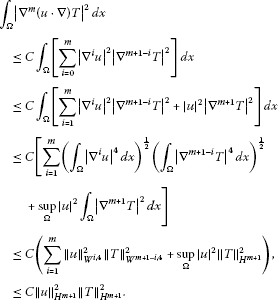Then $\left(u\cdot \mathrm{\nabla }\right)T\in {H}^{m}\left(\mathrm{\Omega }\right)$ and ${F}_{2}\left(\varphi \right)\in {H}^{m}\left(\mathrm{\Omega }\right)$.

We have from the formula (3.1)

${T}_{t}={L}_{2}{\mathrm{\Phi }}_{2}\left(t\right){T}_{0}+{\int }_{0}^{t}{L}_{2}{\mathrm{\Phi }}_{2}\left(t-\tau \right){F}_{2}\left(\mathrm{\Phi }\right)\phantom{\rule{0.2em}{0ex}}d\tau +{F}_{2}\left(\mathrm{\Phi }\right).$

Then there exists α satisfying $0<\alpha <1$ such that

$\begin{array}{rcl}{\parallel {T}_{t}\parallel }_{{H}^{m-\alpha }}& =& {\parallel {L}_{2}{\mathrm{\Phi }}_{2}\left(t\right){T}_{0}+{\int }_{0}^{t}{L}_{2}{\mathrm{\Phi }}_{2}\left(t-\tau \right){F}_{2}\left(\mathrm{\Phi }\right)\phantom{\rule{0.2em}{0ex}}d\tau +{F}_{2}\left(\mathrm{\Phi }\right)\parallel }_{{H}^{m-\alpha }}\\ \le & {\parallel {L}_{2}{\mathrm{\Phi }}_{2}\left(t\right){T}_{0}\parallel }_{{H}^{m-\alpha }}+{\parallel {\int }_{0}^{t}{L}_{2}{\mathrm{\Phi }}_{2}\left(t-\tau \right){F}_{2}\left(\mathrm{\Phi }\right)\phantom{\rule{0.2em}{0ex}}d\tau \parallel }_{{H}^{m-\alpha }}+{\parallel {F}_{2}\left(\mathrm{\Phi }\right)\parallel }_{{H}^{m-\alpha }}\\ \le & C+{\int }_{0}^{t}{\parallel {L}_{2}^{\frac{2+m-\alpha }{2}}{\mathrm{\Phi }}_{2}\left(t-\tau \right){F}_{2}\left(\mathrm{\Phi }\right)\parallel }_{{L}^{2}}\phantom{\rule{0.2em}{0ex}}d\tau \\ \le & C+{\int }_{0}^{t}\parallel {L}_{2}^{1-\frac{\alpha }{2}}{\mathrm{\Phi }}_{2}\left(t-\tau \right)\parallel {\parallel {L}_{2}^{\frac{m}{2}}{F}_{2}\left(\mathrm{\Phi }\right)\parallel }_{{L}^{2}}\phantom{\rule{0.2em}{0ex}}d\tau \le C,\end{array}$

which implies ${T}_{t}\in {H}^{m-\alpha }\left(\mathrm{\Omega }\right)$. Then ${f}_{1}\in {H}^{m-\alpha }\left(\mathrm{\Omega }\right)$ in Eq. (4.8). It follows from the linear elliptic equation $T\in {H}^{m+2-\alpha }\left(\mathrm{\Omega }\right)$ that $\left(u\cdot \mathrm{\nabla }\right)T\in {H}^{m+1-\alpha }\left(\mathrm{\Omega }\right)$ and ${F}_{2}\left(\varphi \right)\in {H}^{m+1-\alpha }\left(\mathrm{\Omega }\right)$. We obtain

$\begin{array}{rcl}{\parallel {T}_{t}\parallel }_{{H}^{m}}& =& {\parallel {L}_{2}{\mathrm{\Phi }}_{2}\left(t\right){T}_{0}+{\int }_{0}^{t}{L}_{2}{\mathrm{\Phi }}_{2}\left(t-\tau \right){F}_{2}\left(\mathrm{\Phi }\right)\phantom{\rule{0.2em}{0ex}}d\tau +{F}_{2}\left(\mathrm{\Phi }\right)\parallel }_{{H}^{m}}\\ \le & {\parallel {L}_{2}{\mathrm{\Phi }}_{2}\left(t\right){u}_{0}\parallel }_{{H}^{m}}+{\parallel {\int }_{0}^{t}{L}_{2}{\mathrm{\Phi }}_{2}\left(t-\tau \right){F}_{2}\left(\mathrm{\Phi }\right)\phantom{\rule{0.2em}{0ex}}d\tau \parallel }_{{H}^{m}}+{\parallel {F}_{2}\left(\mathrm{\Phi }\right)\parallel }_{{H}^{m}}\\ \le & C+{\int }_{0}^{t}{\parallel {L}_{2}^{1+\frac{m}{2}}{\mathrm{\Phi }}_{2}\left(t-\tau \right){F}_{2}\left(\mathrm{\Phi }\right)\parallel }_{{L}^{2}}\phantom{\rule{0.2em}{0ex}}d\tau \\ \le & C+{\int }_{0}^{t}\parallel {L}_{2}^{\frac{1+\alpha }{2}}{\mathrm{\Phi }}_{2}\left(t-\tau \right)\parallel {\parallel {L}_{2}^{\frac{m+1-\alpha }{2}}{F}_{2}\left(\mathrm{\Phi }\right)\parallel }_{{L}^{2}}\phantom{\rule{0.2em}{0ex}}d\tau \le C.\end{array}$

Then ${T}_{t}\in {H}^{m}\left(\mathrm{\Omega }\right)$. We have ${f}_{1}\in {H}^{m}\left(\mathrm{\Omega }\right)$ in Eq. (4.8). Then $T\in {H}^{m+2}\left(\mathrm{\Omega }\right)$ from the theory of linear elliptic equations. Thus,

$T\in {L}^{\mathrm{\infty }}\left(\left(0,T\right),{H}^{m+2}\left(\mathrm{\Omega }\right)\right).$

From the formula (3.1), we induce

$T\in C\left(\left[0,T\right],{H}^{m+2}\left(\mathrm{\Omega }\right)\right),\phantom{\rule{1em}{0ex}}{u}_{t}\in C\left(\left(0,T\right],{H}^{m}\left(\mathrm{\Omega }\right)\right).$

Similarly,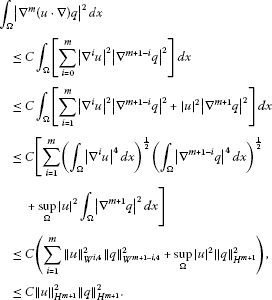Then $\left(u\cdot \mathrm{\nabla }\right)q\in {H}^{m}\left(\mathrm{\Omega }\right)$ and ${F}_{3}\left(\varphi \right)\in {H}^{m}\left(\mathrm{\Omega }\right)$.

We have from the formula (3.1)

${q}_{t}={L}_{3}{\mathrm{\Phi }}_{3}\left(t\right){q}_{0}+{\int }_{0}^{t}{L}_{3}{\mathrm{\Phi }}_{3}\left(t-\tau \right){F}_{3}\left(\mathrm{\Phi }\right)\phantom{\rule{0.2em}{0ex}}d\tau +{F}_{3}\left(\mathrm{\Phi }\right).$

Then there exists α satisfying $0<\alpha <1$ such that

$\begin{array}{rcl}{\parallel {q}_{t}\parallel }_{{H}^{m-\alpha }}& =& {\parallel {L}_{3}{\mathrm{\Phi }}_{3}\left(t\right){q}_{0}+{\int }_{0}^{t}{L}_{3}{\mathrm{\Phi }}_{3}\left(t-\tau \right){F}_{3}\left(\mathrm{\Phi }\right)\phantom{\rule{0.2em}{0ex}}d\tau +{F}_{3}\left(\mathrm{\Phi }\right)\parallel }_{{H}^{m-\alpha }}\\ \le & {\parallel {L}_{3}{\mathrm{\Phi }}_{3}\left(t\right){u}_{0}\parallel }_{{H}^{m-\alpha }}+{\parallel {\int }_{0}^{t}{L}_{3}{\mathrm{\Phi }}_{3}\left(t-\tau \right){F}_{3}\left(\mathrm{\Phi }\right)\phantom{\rule{0.2em}{0ex}}d\tau \parallel }_{{H}^{m-\alpha }}+{\parallel {F}_{3}\left(\mathrm{\Phi }\right)\parallel }_{{H}^{m-\alpha }}\\ \le & C+{\int }_{0}^{t}{\parallel {L}_{3}^{\frac{2+m-\alpha }{2}}{\mathrm{\Phi }}_{3}\left(t-\tau \right){F}_{3}\left(\mathrm{\Phi }\right)\parallel }_{{L}^{2}}\phantom{\rule{0.2em}{0ex}}d\tau \\ \le & C+{\int }_{0}^{t}\parallel {L}_{3}^{1-\frac{\alpha }{2}}{\mathrm{\Phi }}_{3}\left(t-\tau \right)\parallel {\parallel {L}_{3}^{\frac{m}{2}}{F}_{3}\left(\mathrm{\Phi }\right)\parallel }_{{L}^{2}}\phantom{\rule{0.2em}{0ex}}d\tau \le C,\end{array}$

which implies ${q}_{t}\in {H}^{m-\alpha }\left(\mathrm{\Omega }\right)$. Then ${f}_{2}\in {H}^{m-\alpha }\left(\mathrm{\Omega }\right)$ in Eq. (4.11). Thus, $q\in {H}^{m+2-\alpha }\left(\mathrm{\Omega }\right)$ from the theory of linear elliptic equations. Then $\left(u\cdot \mathrm{\nabla }\right)q\in {H}^{m+1-\alpha }\left(\mathrm{\Omega }\right)$ and ${F}_{3}\left(\varphi \right)\in {H}^{m+1-\alpha }\left(\mathrm{\Omega }\right)$. Thus,

$\begin{array}{rcl}{\parallel {q}_{t}\parallel }_{{H}^{m}}& =& {\parallel {L}_{3}{\mathrm{\Phi }}_{3}\left(t\right){q}_{0}+{\int }_{0}^{t}{L}_{3}{\mathrm{\Phi }}_{3}\left(t-\tau \right){F}_{3}\left(\mathrm{\Phi }\right)\phantom{\rule{0.2em}{0ex}}d\tau +{F}_{3}\left(\mathrm{\Phi }\right)\parallel }_{{H}^{m}}\\ \le & {\parallel {L}_{3}{\mathrm{\Phi }}_{3}\left(t\right){q}_{0}\parallel }_{{H}^{m}}+{\parallel {\int }_{0}^{t}{L}_{3}{\mathrm{\Phi }}_{3}\left(t-\tau \right){F}_{3}\left(\mathrm{\Phi }\right)\phantom{\rule{0.2em}{0ex}}d\tau \parallel }_{{H}^{m}}+{\parallel {F}_{3}\left(\mathrm{\Phi }\right)\parallel }_{{H}^{m}}\\ \le & C+{\int }_{0}^{t}{\parallel {L}_{3}^{1+\frac{m}{2}}{\mathrm{\Phi }}_{3}\left(t-\tau \right){F}_{3}\left(\mathrm{\Phi }\right)\parallel }_{{L}^{2}}\phantom{\rule{0.2em}{0ex}}d\tau \\ \le & C+{\int }_{0}^{t}\parallel {L}_{3}^{\frac{1+\alpha }{2}}{\mathrm{\Phi }}_{3}\left(t-\tau \right)\parallel {\parallel {L}_{3}^{\frac{m+1-\alpha }{2}}{F}_{3}\left(\mathrm{\Phi }\right)\parallel }_{{L}^{2}}\phantom{\rule{0.2em}{0ex}}d\tau \le C,\end{array}$

which implies ${q}_{t}\in {H}^{m}\left(\mathrm{\Omega }\right)$. We find ${f}_{2}\in {H}^{m}\left(\mathrm{\Omega }\right)$ in Eq. (4.11). Then $q\in {H}^{m+2}\left(\mathrm{\Omega }\right)$ from the theory of linear elliptic equations. We have

$q\in {L}^{\mathrm{\infty }}\left(\left(0,T\right),{H}^{m+2}\left(\mathrm{\Omega }\right)\right).$

From the formula (3.1), we see

$q\in C\left(\left[0,T\right],{H}^{m+2}\left(\mathrm{\Omega }\right)\right),\phantom{\rule{2em}{0ex}}{q}_{t}\in C\left(\left(0,T\right],{H}^{m}\left(\mathrm{\Omega }\right)\right).$

It follows from Eq. (4.5) that

$\mathrm{\nabla }p=\mathrm{\Delta }u-\sigma u+\left(RT-\stackrel{˜}{R}q\right)\stackrel{\to }{\kappa }-\frac{1}{Pr}\left(u\cdot \mathrm{\nabla }\right)u-{u}_{t}.$

Clearly, the right-hand side of the above equality is continuous in ${H}^{m}\left(\mathrm{\Omega }\right)$. Then

$p\in C\left(\left(0,T\right),{H}^{m+1}\left(\mathrm{\Omega }\right)\right).$

The proof is completed. □

Since the differentiability of time and of space can be transformed into each other, we obtain

Remark 4.3 If ${\varphi }_{0}=\left({u}_{0},{T}_{0},{q}_{0}\right)\in {H}^{k+2}\left(\mathrm{\Omega },{R}^{4}\right)\cap {H}_{1}$, $Q,G\in {H}^{k}\left(\mathrm{\Omega }\right)$, then Eqs. (1.1)-(1.7) have a higher regular solution $\left(\varphi ,p\right)=\left(u,T,q,p\right)$, and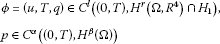for $\mathrm{\forall }0, where l, r, α, β are positive integers satisfying $2l+r=k+2$ and $2\alpha +\beta =k+1$.

## References

1. Luo, H: Global solution of atmospheric circulation equations with humidity effect (submitted)

2. Ma T, Wang SH: Phase Transition Dynamics in Nonlinear Sciences. Springer, New York; 2012.

3. Ma T: Theories and Methods in Partial Differential Equations. Science Press, China; 2011. in Chinese

4. Phillips NA: The general circulation of the atmosphere: a numerical experiment. Q. J. R. Meteorol. Soc. 1956, 82: 123-164. 10.1002/qj.49708235202

5. Rossby CG: On the solution of problems of atmospheric motion by means of model experiment. Mon. Weather Rev. 1926, 54: 237-240. 10.1175/1520-0493(1926)54<237:OTSOPO>2.0.CO;2

6. Lions JL, Temam R, Wang SH: New formulations of the primitive equations of atmosphere and applications. Nonlinearity 1992, 5(2):237-288. 10.1088/0951-7715/5/2/001

7. Lions JL, Temam R, Wang SH: On the equations of the large-scale ocean. Nonlinearity 1992, 5(5):1007-1053. 10.1088/0951-7715/5/5/002

8. Lions JL, Temam R, Wang SH: Models for the coupled atmosphere and ocean. (CAO I,II). Comput. Mech. Adv. 1993, 1(1):5-54.

9. Luo H: Steady state solution to atmospheric circulation equations with humidity effect. J. Appl. Math. 2012. doi:10.1155/2012/867310

10. Evens LC: Partial Differential Equations. Am. Math. Soc., Providence; 1998.

11. Temam R CBMS-NSF Regional Conference Series in Applied Mathematics. In Navier-Stokes Equation and Nonlinear Functional Analysis. SIAM, Philadelphia; 1983.

12. Temam R: Navier-Stokes Equations: Theory and Numerical Analysis. North-Holland, Amsterdam; 1979.

13. Pazy A Appl. Math. Sci. 44. In Semigroups of Linear Operators and Applications to Partial Differential Equations. Springer, Berlin; 2006.

14. Ma T, Wang SH: Stability and Bifurcation of Nonlinear Evolution Equations. Science Press, China; 2007. in Chinese

15. Ma T, Wang SH Nonlinear Science Ser. A 53. In Bifurcation Theory and Applications. World Scientific, Singapore; 2005.

16. Temam R Applied Mathematical Science 68. In Infinite-Dimensional Dynamical Systems in Mechanics and Physics. 2nd edition. Springer, New York; 1997.

## Acknowledgements

The author is very grateful to the anonymous referees whose careful reading of the manuscript and valuable comments enhanced presentation of the manuscript. The project is supported by the National Natural Science Foundation of China (11271271), the NSF of Sichuan Science and Technology Department of China (2010JY0057) and the NSF of Sichuan Education Department of China (11ZA102).

## Author information

Authors

### Corresponding author

Correspondence to Hong Luo.

### Competing interests

The author declares that he has no competing interests.

## Rights and permissions

Reprints and Permissions

Luo, H. Regularity of global solution to atmospheric circulation equations with humidity effect. Bound Value Probl 2012, 143 (2012). https://doi.org/10.1186/1687-2770-2012-143

• Accepted:

• Published:

• DOI: https://doi.org/10.1186/1687-2770-2012-143

### Keywords

• global solution
• regularity
• atmospheric circulation equations
• humidity effect